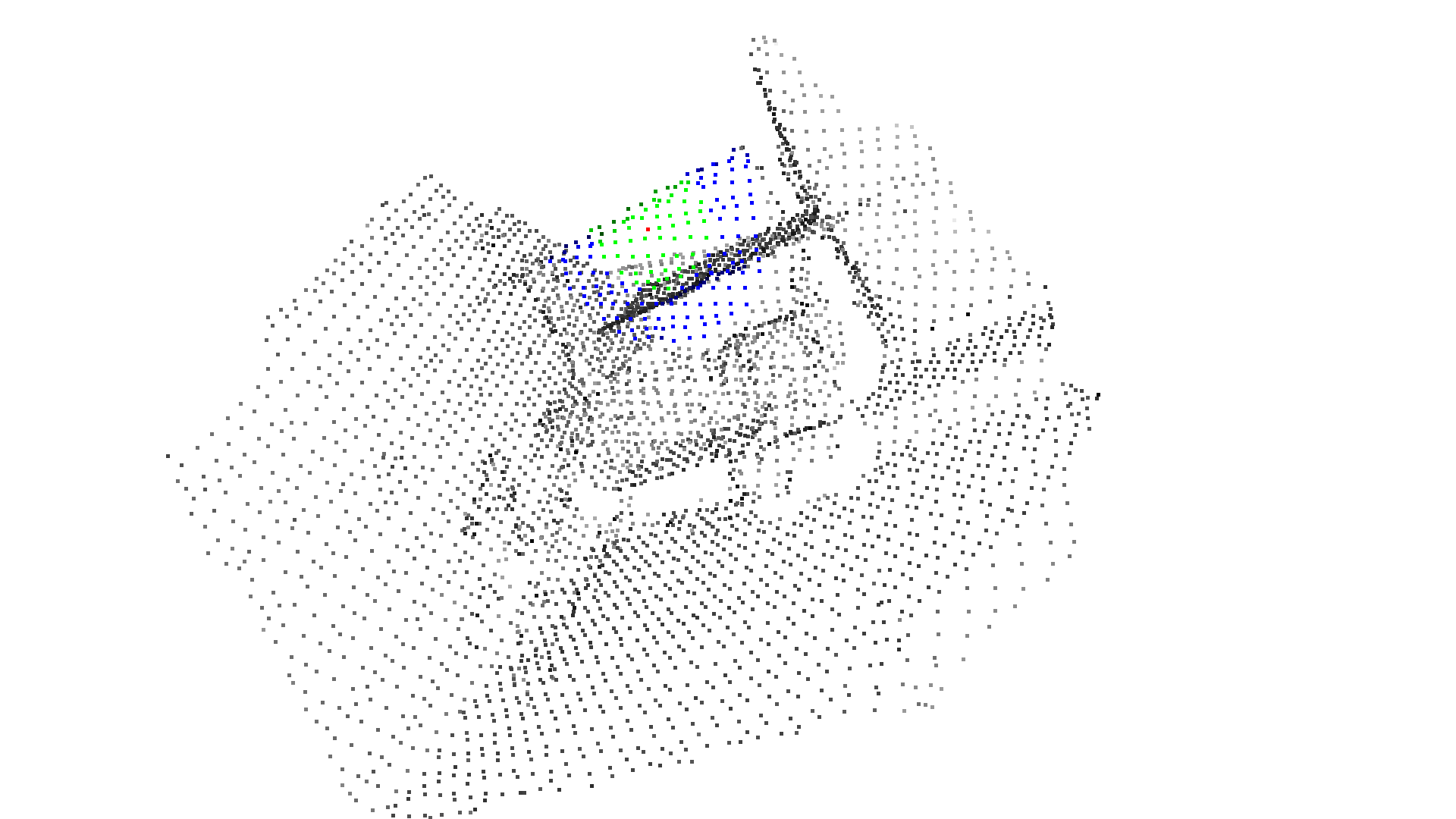# KDTree¶

Open3D uses FLANN to build KDTrees for fast retrieval of nearest neighbors.

## Build KDTree from point cloud¶

The code below reads a point cloud and builds a KDTree. This is a preprocessing step for the following nearest neighbor queries.

:

print("Testing kdtree in Open3D...")
print("Load a point cloud and paint it gray.")
pcd.paint_uniform_color([0.5, 0.5, 0.5])
pcd_tree = o3d.geometry.KDTreeFlann(pcd)

Testing kdtree in Open3D...
Load a point cloud and paint it gray.


## Find neighboring points¶

We pick the 1500th point as the anchor point and paint it red.

:

print("Paint the 1500th point red.")
pcd.colors = [1, 0, 0]

Paint the 1500th point red.


### Using search_knn_vector_3d¶

The function search_knn_vector_3d returns a list of indices of the k nearest neighbors of the anchor point. These neighboring points are painted with blue color. Note that we convert pcd.colors to a numpy array to make batch access to the point colors, and broadcast a blue color [0, 0, 1] to all the selected points. We skip the first index since it is the anchor point itself.

:

print("Find its 200 nearest neighbors, and paint them blue.")
[k, idx, _] = pcd_tree.search_knn_vector_3d(pcd.points, 200)
np.asarray(pcd.colors)[idx[1:], :] = [0, 0, 1]

Find its 200 nearest neighbors, and paint them blue.


Similarly, we can use search_radius_vector_3d to query all points with distances to the anchor point less than a given radius. We paint these points with a green color.

:

print("Find its neighbors with distance less than 0.2, and paint them green.")
[k, idx, _] = pcd_tree.search_radius_vector_3d(pcd.points, 0.2)
np.asarray(pcd.colors)[idx[1:], :] = [0, 1, 0]

Find its neighbors with distance less than 0.2, and paint them green.

:

print("Visualize the point cloud.")
o3d.visualization.draw_geometries([pcd],
zoom=0.5599,
front=[-0.4958, 0.8229, 0.2773],
lookat=[2.1126, 1.0163, -1.8543],
up=[0.1007, -0.2626, 0.9596])

Visualize the point cloud.Note:

Besides the KNN search search_knn_vector_3d and the RNN search search_radius_vector_3d, Open3D provides a hybrid search function search_hybrid_vector_3d. It returns at most k nearest neighbors that have distances to the anchor point less than a given radius. This function combines the criteria of KNN search and RNN search. It is known as RKNN search in some literatures. It has performance benefits in many practical cases, and is heavily used in a number of Open3D functions.